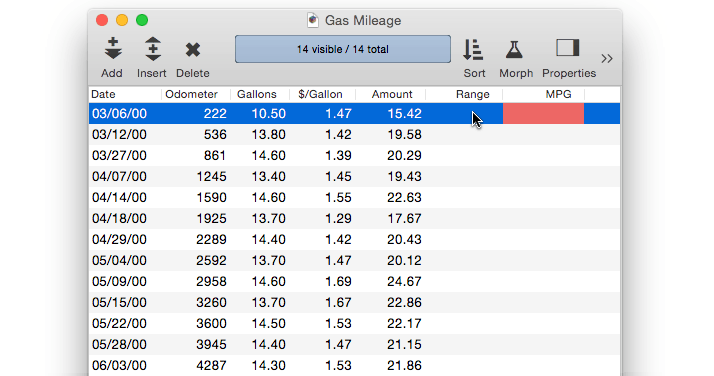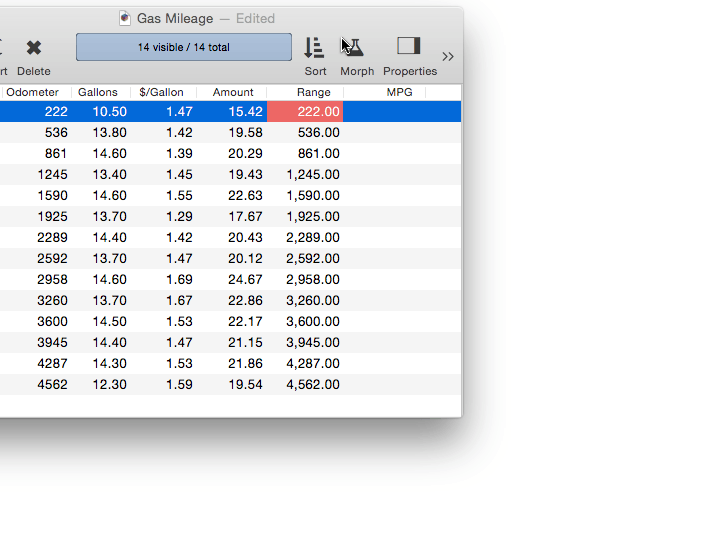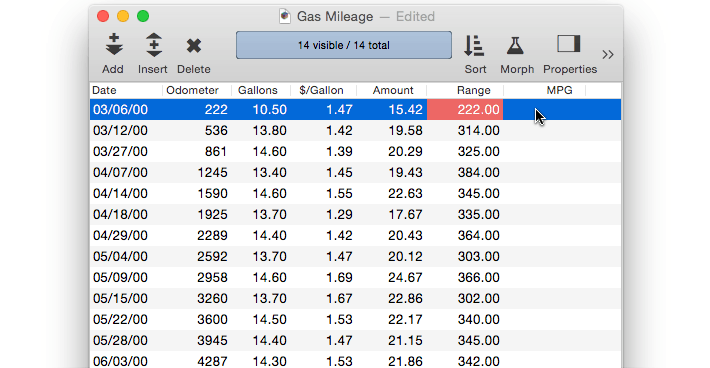Running Difference is the opposite of Running Total. Running Difference fills each data cell with the difference between the cell and the cell above it. Use Running Difference when you want to compute the spread or interval between consecutive values, for example odometer readings or dates.

If you use the Running Difference command on your raw data, the raw data will be destroyed in the process of calculating the running difference. We recommend that you avoid this problem by creating an extra field to hold the running difference. You can use the Morph Field Dialog to copy the data into the field, and then use the Running Difference command without disturbing the original raw data.

#### Using Running Difference to Calculate Gas Mileage

Let’s take a look at how to calculate your gas mileage using the Running Difference command. Start with empty Range and MPG fields, then use the Morph Field Dialog to copy the Odometer field into the Range field.Now use the Running Difference command (right-click on the field name and select Math>Running Difference) to convert the odometer readings into the distance between fillups.Now we’ll calculate the actual miles per gallon. Move to the MPG field and use the Morph Field Dialog to calculate the formula Range/Gallons. Presto, the miles per gallon between each fill-up is calculated.You’ll need to repeat these steps periodically as you continue to drive. This process can be automated with a procedure.

``````field Range
formulafill Odometer
runningdifference
field MPG
formulafill Range/Gallons
``````